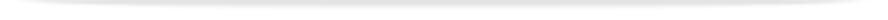units

MEC2456

Faculty of Engineeringprint version

This unit entry is for students who completed this unit in 2016 only. For students planning to study the unit, please refer to the unit indexes in the the current edition of the Handbook. If you have any queries contact the managing faculty for your course or area of study.

MEC2456 - Engineering computational analysis

6 points, SCA Band 2, 0.125 EFTSL

Refer to the specific census and withdrawal dates for the semester(s) in which this unit is offered.

Faculty

Engineering

Organisational Unit

Department of Mechanical and Aerospace Engineering

Coordinator(s)

Dr Zhe Liu (Clayton); Dr Ooi Ean Hin (Malaysia)

Offered

Clayton

• Second semester 2016 (Day)

Malaysia

• Second semester 2016 (Day)

Synopsis

This unit conveys the fundamentals of numerical analysis techniques for root-finding, interpolation, integration, the solution of ordinary differential equations and data analysis, and Matlab is employed to demonstrate their implementation. The role computers play in both the solution of engineering problems and the acquisition and analysis of data is explored through consideration of common partial differential equations in mechanics, and their solution via finite difference, finite volume, and finite element methods. Exposure to commercial finite-element analysis and computational fluid dynamics codes provides experience in solving practical engineering problems.

Outcomes

1. understand the role of computers and numerical analysis in modern engineering practice
2. ability to evaluate stability, efficiency and accuracy constraints on available methods for numerical approximation of engineering solutions
3. ability to apply numerical methods for interpolation, root-finding, integration, solution of ordinary and partial differential equations, and analysis of data.
4. knowledge and skills to generate accurate solutions to engineering problems using numerical computing
5. knowledge of the types of equations which arise in computational mechanics
6. understanding of the use of finite difference, finite volume and finite element methods, to solve computational mechanics problems
7. understanding and applying methods for data analysis, including sampling, Fourier transforms and filteringSolve engineering problems numerically
8. determine the appropriate technique to solve a problem through consideration of the accuracy, efficiency and stability of available methods
9. acquire, analyse and interpret data
10. complete tasks as part of a teamImprove oral and written communication skills
11. appreciation of the role of computers in engineering industry
12. confidence in identifying engineering problems and formulating original solutions

Assessment

Laboratory and Assignments: 30%
Examination (3 hours): 70%
Students are required to achieve at least 45% in the total continuous assessment component and at least 45% in the final examination component and an overall mark of 50% to achieve a pass grade in the unit. Students failing to achieve this requirement will be given a maximum of 45% in the unit.

3 hour lectures, 2 hours practice sessions or laboratories per week and 6 hours of private study per week

None

Prohibitions

None